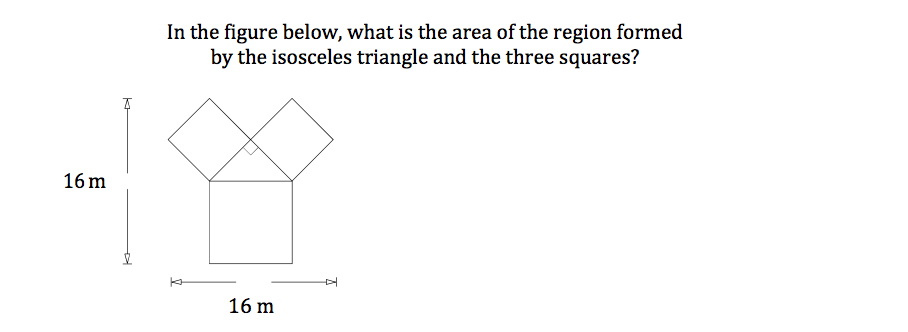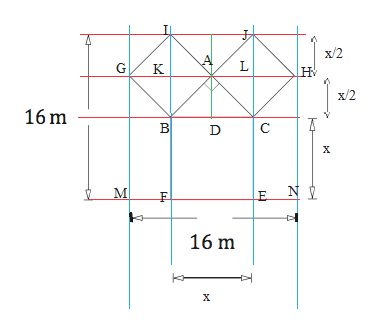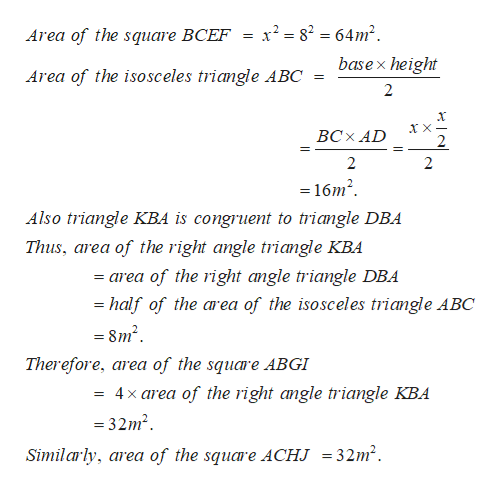# In the figure below, what is the area of the region formedby the isosceles triangle and the three squares?16 m16 m

Question
1 viewshelp_outlineImage TranscriptioncloseIn the figure below, what is the area of the region formed by the isosceles triangle and the three squares? 16 m 16 m fullscreen
check_circle

Step 1

Firstly, let us name the points as shown below:help_outlineImage Transcriptioncloseх/2 н G К х/2 16 m В С х NI М Е 16 m х fullscreen
Step 2

Now, calculating the ar...help_outlineImage Transcriptionclose282 64m2 Area of the square BCEF basex height Area of the isosceles triangle ABC 2 ВСх AD 2 = 2 2 -16m2 Also triangle KBA is congruent to triangle DBA Thus, area of the right angle triangle KBA = area of the right angle triangle DBA -half of the area of the isosceles triangle ABC = 8m2 Therefore, area of the square ABGI 4 x area of the right angle triangle KBA =32m2 Similarly area of the square ACHJ = 32m2 fullscreen

### Want to see the full answer?

See Solution

#### Want to see this answer and more?

Solutions are written by subject experts who are available 24/7. Questions are typically answered within 1 hour.*

See Solution
*Response times may vary by subject and question.
Tagged in

### Calculus